EP0492565B1 - Apparatus for calculating geometrical view factor - Google Patents

Info

Publication number
EP0492565B1
EP0492565B1 EP91122079A EP91122079A EP0492565B1 EP 0492565 B1 EP0492565 B1 EP 0492565B1 EP 91122079 A EP91122079 A EP 91122079A EP 91122079 A EP91122079 A EP 91122079A EP 0492565 B1 EP0492565 B1 EP 0492565B1
Authority
EP
European Patent Office
Prior art keywords
calculating
angle
factor
energy
intensity
Prior art date
Legal status (The legal status is an assumption and is not a legal conclusion. Google has not performed a legal analysis and makes no representation as to the accuracy of the status listed.)
Application number
EP91122079A
Other languages
German (de)
French (fr)
Other versions
EP0492565A2 (en
EP0492565A3 (en
Inventor
Yoshihiro Hattori
Sachio Rune-Fujinomori 609 Nagamitsu
Yoichi Nagata
Hisashi Kodama
Current Assignee (The listed assignees may be inaccurate. Google has not performed a legal analysis and makes no representation or warranty as to the accuracy of the list.)
Panasonic Corp
Original Assignee
Panasonic Corp
Priority date (The priority date is an assumption and is not a legal conclusion. Google has not performed a legal analysis and makes no representation as to the accuracy of the date listed.)
Filing date
Publication date
Priority to JP41622690 priority Critical
Priority to JP416226/90 priority
Priority to JP51001/91 priority
Priority to JP5100091 priority
Priority to JP51000/91 priority
Priority to JP5100191 priority
Application filed by Panasonic Corp filed Critical Panasonic Corp
Publication of EP0492565A2 publication Critical patent/EP0492565A2/en
Publication of EP0492565A3 publication Critical patent/EP0492565A3/xx
Application granted granted Critical
Publication of EP0492565B1 publication Critical patent/EP0492565B1/en
Anticipated expiration legal-status Critical
Expired - Lifetime legal-status Critical Current

• 230000000875 corresponding Effects 0.000 claims description 10
• 230000000414 obstructive Effects 0.000 claims description 4
• 238000010438 heat treatment Methods 0.000 description 11
• 239000007787 solids Substances 0.000 description 8
• 238000004364 calculation methods Methods 0.000 description 6
• 230000005540 biological transmission Effects 0.000 description 5
• 238000010276 construction Methods 0.000 description 3
• 238000010586 diagrams Methods 0.000 description 3
• 280000606094 Magazine companies 0.000 description 2
• 239000003570 air Substances 0.000 description 2
• 238000007796 conventional methods Methods 0.000 description 2
• 238000001816 cooling Methods 0.000 description 2
• 280000125387 Around The Point companies 0.000 description 1
• 238000000342 Monte Carlo simulations Methods 0.000 description 1
• 241001417517 Scatophagidae Species 0.000 description 1
• 238000004378 air conditioning Methods 0.000 description 1

Images

•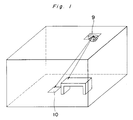••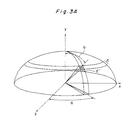•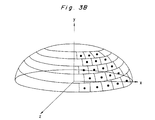•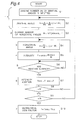•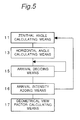•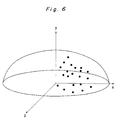•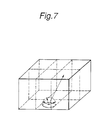•Classifications

• GPHYSICS
• G06COMPUTING; CALCULATING; COUNTING
• G06FELECTRIC DIGITAL DATA PROCESSING
• G06F30/20Design optimisation, verification or simulation
• G06F30/23Design optimisation, verification or simulation using finite element methods [FEM] or finite difference methods [FDM]

Description

BACKGROUND OF THE INVENTION
The present invention relates to an apparatus for calculating a geometrical view factor in performing a calculation about radiant heat.
The present invention relates to an apparatus for calculating the geometrical view factor of a rectangular surface required in performing a calculation quickly and accurately about radiation heat in a three-dimensional region.
In recent years, radiant air conditioners, for example, a floor heating apparatus and a panel cooling and heating of a ceiling has been developed. In order to develop this kind of floor heating apparatus, it is necessary to know the magnitude of radiant heat applied to the wall of a room in which the floor heating apparatus is installed. In order to calculate the radiant heat, it is necessary to find a geometrical view factor which depends on the angular or positional relationship between the floor heating apparatus and an object, for example, the wall of the room. As a method for finding the geometrical view factor, energy lines are emitted from a point of the floor heating apparatus to a wall to be radiantly heated and the number of energy lines which have reached the wall are counted.
A conventional apparatus for calculating the geometrical view factor is constructed as shown in Fig. 5 and represents the closest prior art from which the invention proceeds. The operation of the apparatus is described below. A hemispherical surface is assumed around the point of the floor heating apparatus. Zenithal angle calculating means 11 generates random numbers in the zenithal angle direction of the spherical surface to find a zenithal angle of the spherical surface. Horizontal angle calculating means 13 generates random numbers in the horizontal angle direction of the spherical surface to find a horizontal angle of the spherical surface. Arrival deciding means 15 decides whether or not an energy line having the decided zenithal angle and horizontal angle reaches a surface of an object. Based on the number of emitted energy lines, arrival intensity adding means 16 calculates the intensities of energy lines at the surface which they have reached. Geometrical view factor calculating means 17 finds the ratio of the intensities of the energy lines at the surface to the intensities of all emitted energy lines to find a geometrical view factor. Refer to a magazine entitled as "Thermal Radiation View Factor Calculation Using Monte Carlo Method, Comparison of Accuracy and Computer Time with Area Integration Method" written by Takeshi IKUSHIMA and et al. and published by Japan Atomic Society, 1988, Vol. 30, No. 6, page 64 - page 70.
Fig. 6 is an image view showing a method for generating energy lines by utilizing random numbers so as to calculate the number of energy lines which have reached each surface.
However, according to the above-described conventional method, since random numbers are utilized, energy lines emitted from a certain point of an energy source are not necessarily emitted isotropically toward a hemisphere, which means that the number of energy lines cannot be accurately calculated when a small number of energy lines are emitted from the emission point. If many energy lines are emitted therefrom, it takes long to perform a calculation.
According to the conventional method, a geographical view factor between rectangular surfaces is found as follows: The rectangular surfaces of a three-dimensional region are divided as shown in Fig. 7; energy lines each having an intensity are emitted from a divided rectangular surface of the floor of the region energy source in various directions; the intensity of each energy line which has reached an object rectangular surface of a solid wall of the region is calculated; and the ratio of the intensities of energy lines which have reached the object rectangular surface of the solid wall to the intensity obtained by adding the intensities of all energy lines emitted from the divided rectangular surface is found and then the geometrical view factor between the divided rectangular surface of the floor and the object rectangular surface of the solid wall is founded (Papers of lectures given at meeting of Air Conditioning Sanitary Engineering Society, 1990, page 1161 through 1164 N.Takeya et al.: "Study about numerical estimate of airflow and thermal distribution in room"). Supposing that the emitted energy lines reach the object rectangular surface of the solid wall directly, arrival positions of energy lines are calculated.
However, according to the above ccnventional method, in a space such as a room accommodating a furniture or a furnishing as shown in Fig. 8, an accurate geographical view factor cannot be obtained because it is assumed that the emitted energy lines directly reach the surface of the solid wall and then the existence of the furniture is not considered. In analyzing hot air flow in the room, the space is divided with fine mesh.
In order to quickly trace an energy line in the space which is regarded as not absorbing radiation heat, it is necessary to regenerate meshes by an element which constitutes a solid body which absorbs radiant heat.
When the transmission of radiant heat from air conditioning equipments such as a floor heating equipment, a panel cooling and heating of a ceiling, or the like is calculated, it is necessary to find a geographical view factor which is determined according to an angle and a position. Specially, it is necessary to find a geographical view factor between a human body and a rectangular surface in order to calculate the transmission of radiant heat between the human body and a radiant wall. In such calculations, a model is considered as the human body in order to perform the calculations. Conventionally, the human body is regarded as a microelement to find the geographical view factor between the micro-cylinder and the rectangular surface as shown in Fig. 9. This method is described in a magazine of "Ann. Physiological Anthrop." published in 1988, in 7(3), on pages 143 through 150: T. Horikoshi et.al.: "Configuration Factors between Cylindrical Element as a Model of the Human Body and a Rectangular Plane". In order to find the geographical view factor, an area integration equation is used by finely dividing the cylindrical surface and the rectangular surface.
However, according to the conventional art, it takes a long period of time for calculations to be performed because cylindrical and rectangular surfaces must be finely divided. In addition, it is necessary to limit an integration range if another rectangular surface is interposed between the cylindrical surface and the rectangular surface. Thus, accurate calculations cannot be performed. Further, in performing calculation, attention is not paid to the actual dimensions of the human body. Therefore, the influence of radiant heat from the rectangular surface to the human body is not correctly calculated.
SUMMARY OF THE INVENTION
It is an object of the present invention to provide an apparatus for calculating a thermal radiant geometrical view factor accurately and quickly.
It is an object of the present invention to provide an apparatus for tracing emitted energy lines accurately and quickly.
In accomplishing these and other objects, according to the present invention, there is provided an apparatus for calculating a geometrical view factor for radiant heat determination between an object surface and a rectangular surface serving as a bottom surface of a spherical surface assumed on the rectangular surface when energy lines are emitted from a center of the bottom surface of the spherical surface on the rectangular surface to the object surface, comprising:
• first calculating means for calculating a zenithal angle of each energy line by dividing the spherical surface in a zenithal angle direction at equal intervals;
• second calculating means for calculating a dividing number of horizontal angles corresponding to the zenithal angle calculated by the first calculating means;
• third calculating means for calculating a divided horizontal angle by dividing an entire horizontal angle of the spherical surface into the dividing number of horizontal angles determined by the second calculating means;
• fourth calculating means for calculating an intensity corresponding to the zenithal angle determined by the third calculating means;
• input means for inputting a coordinate of a configuration of an obstruction for preventing the emitted energy line from reaching the object surface;
• deciding means for deciding whether or not the energy lines emitted from the center of the bottom surface the spherical surface at the intensity calculated by the fourth calculating means reach the object surface while the energy lines pass through a point on the spherical surface corresponding to the zenithal angle and the horizontal angle determined by the first and third calculating means, based on the coordinated of the obstruction inputted by the inputting means;
• adding means for adding to each other the intensities of the energy lines which have reached each object surface based on a decision made by the deciding means; and
• means for calculating the geometrical view factor by dividing by the intensity of the entire energy lines the intensity of each object surface obtained by the addition performed by the adding means.
• According to such apparatus, the zenithal angle of a spherical surface is divided at regular intervals, the horizontal angle thereof is also divided at regular intervals in correspondence with each of the zenithal angles, and in addition, the intensity of each emitted energy line corresponds to each zenithal angle. Thus, the energy lines can be isotropically emitted from the center of the bottom surface of the spherical surface. The emitted energy lines are traced from the emission point to each object surface and the intensity of each energy line which has reached each object surface are added to each other. Then, the intensity obtained by the addition is divided by the intensity of entire energy lines emitted from the emission point. Thus, the geometrical view factor of each surface can be found quickly and accurately.
BRIEF DESCRIPTION OF THE DRAWINGS
These and other objects and features of the present invention will become apparent from the following description taken in conjunction with the preferred embodiments thereof with reference to the accompanying drawings, in which:
• Fig. 1 is a perspective view showing an indoor space to find a geometrical view factor according to a preferred embodiment of the present invention;
• Fig. 2 is a block diagram showing the construction of an apparatus for calculating a geometrical view factor;
• Fig. 3A is a schematically perspective view showing the operation for dividing the hemispherical surface in the apparatus of Fig. 2:
• Fig. 3B is a schematically perspective view showing the operation for emitting energy lines in the apparatus of Fig. 2;
• Fig. 4 is a flowchart showing the operation of the preferred embodiment;
• Fig. 5 is a block diagram showing the construction of a conventional apparatus for calculating a geometrical view factor;
• Fig. 6 is a schematically perspective view snowing the content of the conventional apparatus for calculating a geometrical view factor;
• Fig. 7 is an illustration showing energy line tracing to be carried out by a conventional apparatus for calculating a geometrical view factor;
• Fig. 8 is an illustration showing an energy line tracing to be carried out by the conventional apparatus when there is a furniture in a space; and
• Fig. 9 is a model view in which a human body is supposed as a microcylinder in a conventional apparatus for calculating a geographical view factor.
• DETAILED DESCRIPTION OF THE INVENTION
Before the description of the present invention proceeds, it is to be noted that like parts are designated by like reference numerals throughout the accompanying drawings.
Fig. 1 is a perspective view showing an indoor space provided to find a geometrical view factor according to an embodiment of the present invention. In this embodiment, an energy source 9 such as a heat source or a light source is provided on the ceiling so that what percentage the energy generated by the energy source reaches a divided object rectangular surface of the floor, namely, the geometrical view factor between the energy source and the object area is calculated. A hemispherical surface is assumed around the emission point of the energy source 9. Fig. 2 is a block diagram showing the construction of an apparatus for calculating the geometrical view factor. The apparatus comprises zenithal angle calculating means 1, means 2 for calculating dividing number of horizontal angle, horizontal angle calculating means 3, emission intensity calculating means 4, indoor configuration coordinate input means 5, arrival deciding means 6, arrival intensity adding means 7, and means 8 for calculating geometrical view factor.
The zenithal angle calculating means 1 determines a zenithal angle of the hemispherical surface by dividing the hemispherical surface in its zenithal angle direction at equal intervals. The horizontal angle calculating means 2 calculates the dividing number of the horizontal angle of the hemispherical surface corresponding to the zenithal angle determined by the zenithal angle calculating means 1. The horizontal angle calculating means 3 calculates the horizontal angle by dividing the horizontal angle into the dividing number of the horizontal angle determined by the horizontal angle calculating means 2. The emission intensity calculating means 4 calculates an intensity corresponding to the zenithal angle determined by the horizontal angle calculating means 3. The indoor configuration coordinate input means 5 inputs the coordinate of the configuration of a room including the position of a furniture such as a desk or furnishings. The deciding means 6 decides whether or not an energy line emitted from the center of the bottom surface the hemispherical surface at the above intensity reaches an object surface corresponding to the zenithal angle and the horizontal angle determined by the zenithal angle calculating means 1 and the horizontal angle calculating means 3, respectively. The intensity adding means 7 adds to each other the intensities of energy lines which have reached each object rectangular surface based on the result obtained by the decision made by the input means 5. The geometrical view factor calculating means 8 calculates a geometrical view factor based on the intensity obtained by the addition performed by the deciding means 6. As shown in Fig. 3A, the zenithal angle (b) is an angle which a perpendicular drawn from an emission point to the hemispherical surface forms with y-axis, and the horizontal angle (a) is an angle which a line emitted from the emission point to a given point on the hemispherical surface forms with z-axis.
Fig. 4 is a flowchart showing the operation of the apparatus. First, at step S1, the dividing number (N) of the zenithal angle is inputted. At step S2, the zenithal angle (b) is found by the following equation (1) supposing that (i) is an integer in the range from 1 to N: b = π/(8N) + π/(4N) * i At step S3, the dividing number (M) of the horizontal angle (a) is found by the following equation (2) as an integer corresponding to the zenithal angle (b): M = INT [4Ncosb]
At step S4, based on the dividing number (M) of the horizontal angle (a), the horizontal angle (a) is found from the following equation (3) supposing that (j) is an integer in the range from 1 to M: a = 2π/M * j
The above calculation is described in terms of the length of a dividing line with reference to Fig. 3A. If the zenithal angle (b) is found, the length (d) of the dividing line in the zenithal angle direction is determined. Then, in order to approximate a length (d') in the horizontal direction to the length (d), the circumference in the horizontal direction formed by connecting points on the hemispherical surface which forms the zenithal angle (b) is divided by the length (d). It is assumed that the quotient is (M). If the length (d) does not exactly divide the circumference, the circumference in the horizontal direction is divided by (M+1), with the circumference (M+1) set as a dividing number in the horizontal direction. Consequently, the quotient (d') is obtained. Alternately, the following method may be performed: If the remainder of the division of the circumference by the length (d) is 0.5 or more, the dividing number is set as (M+1). If the remainder of the division of the circumference by the length (d) is less than 0.5, the dividing number is set as (M). Thus, the length (d') of a line in the horizontal direction dividing the circumference can be found. Thus, the horizontal angle (a) is determined.
At step S5, considering the Lambert's cosine law and that the number of energy lines which have reached each hemispherical surface is expressed as an integer, the intensity (T) of the entire energy lines is corrected by the following equation (4): T = sinb * 4Ncosb/M where M is the dividing number in the horizontal direction actually used.
At step S6, based on the horizontal angle (a), the zenithal angle (b), and the coordinate of the indoor configuration, the three-dimensional coordinate of each energy line is geometrically traced until each energy line reaches the object surface of a solid body. When each energy line has reached the object surface of the solid body, it is decided where the energy line has reached, for example, part of the floor surface, the surface of table, or the like. Then, the intensities (T) of the energy lines which have reached each surface are added to each other based on the following equation (5) at step S7. TWALL = T'WALL + T
In the equation (5), when the intensity (T) of the energy line is firstly found, the value (T'WALL) is zero and then in the equation (5), TWALL = T. Then, when the intensity (T) of the energy line is secondly found, the firstly found intensity value (TWALL) is substituted in the equation (5) as a value (T'WALL) and then the firstly found intensity (T'WALL) and the now found intensity (T) are added to each other to find the new intensity (TWALL).
Then, at step S8, (j) is increased by "1" and then the horizontal angle (a) is changed to carry out the operations of steps S4 through S7 again. At step S9, after (j) takes the value "1" through (M) at step S8, (i) is increased by "1" and then the zenithal angle (b) is changed to perform the operation of steps S2 through S8. At step S10, after (i) takes the value "1" through (N) at step S9, the intensity (TWALL) of each surface obtained by the addition is divided by the entire intensity (TALL) of the emission hemispherical surface so as to find a geometrical view factor (F) from the following equation (6): F = TWALL/TALL
Fig. 3B is an image view showing the content of the above-described embodiment of the present invention. As shown in Fig. 3B, energy lines are emitted uniformly.
Unlike the above embodiment in which the energy source is provided on the ceiling in Fig. 1, the emission point of energy lines is provided on the floor surface in order to find the transmission of heat generated by a floor heating apparatus. To this end, energy lines are emitted from the center of the bottom surface a hemisphere to the hemispherical surface as shown in Fig. 3B. The geometrical view factor can be found based on the intensities of energy lines at each surface which the energy line has reached. In addition, the transmission of heat generated by a radiant panel can be found. That is, energy lines are emitted from a given point of the surface of the panel to a hemisphere with the emission point set as the center of the bottom surface the hemisphere. Similarly to the above-described floor heating apparatus, the geometrical view factor can be found.
The above-described means of the present invention may be carried out by software by using a computer, but may be performed by a specialized hardware circuit having each function.
According to the present invention, the geometrical view factor of a cylinder or a human body and a rectangular surface can be precisely and quickly calculated. Thus, the transmission of radiant heat can be accurately and quickly calculate.

Claims (2)

1. An apparatus for calculating a geometrical view factor for radiant heat between an object surface and a rectangular surface serving as a bottom surface of a spherical surface assumed on the rectangular surface when energy lines are emitted from a center of the bottom surface of the spherical surface on the rectangular surface to the object surface, comprising:
first calculating means (1) for calculating a zenithal angle (b) of each energy line;
third calculating means (3) for calculating a divided horizontal angle (a);
deciding means (6) for deciding whether or not the energy lines emited reach the object surface;
adding means (7) for adding to each other the intensities (T) of the energy lines which have reached each object surface based on a decision made by the deciding means (6); and
means (8) for calculating the geometrical view factor by dividing by the intensity (T) of the entire energy lines the intensity (TWALL) of each object surface obtained by the addition performed by the adding means (7);
characterized in that
said first calculating means (1) is provided for calculating said zenithal angle (b) of each energy line by dividing the spherical surface in a zenithal angle direction at equal intervals;
a second calculating means (2) is provided for calculating a dividing number (M) of horizontal angles (a) corresponding to the zenithal angle calculated by the first calculating means (1);
said third calculating means (3) is provided for calculating said divided horizontal angle by dividing an entire horizontal angle of the spherical surface into the dividing number (M) of horizontal angles (a) determined by the second calculating means (2);
a fourth calculating means (4) is provided for calculating an intensity (T) corresponding to the zenithal angle (b) determined by the third calculating means (3);
an input means (5) is provided for inputting a coordinate of a configuration of an obstruction for preventing the emitted energy line from reaching the object surface; and
said deciding means (6) is provided for deciding whether or not the energy lines emitted from the center of the bottom surface of the spherical surface at the intensity (T) calculated by the fourth calculating means (4) reach the object surface while the energy lines pass through a point on the spherical surface corresponding to the zenithal angle (b) and the horizontal angle (a) determined by the first and third calculating means (1, 3), based on the coordinate of the obstruction inputted by the inputting means (5).
2. The apparatus for calculating a geometrical view factor as defined in claim 1, wherein the spherical surface is a hemispherical surface.
EP91122079A 1990-12-27 1991-12-21 Apparatus for calculating geometrical view factor Expired - Lifetime EP0492565B1 (en)

Priority Applications (6)

Application Number Priority Date Filing Date Title
JP41622690 1990-12-27
JP416226/90 1990-12-27
JP51000/91 1991-03-15
JP5100191 1991-03-15
JP51001/91 1991-03-15
JP5100091 1991-03-15

Publications (3)

Publication Number Publication Date
EP0492565A2 EP0492565A2 (en) 1992-07-01
EP0492565A3 EP0492565A3 (en) 1994-04-20
EP0492565B1 true EP0492565B1 (en) 1998-10-14

Family

Family Applications (1)

Application Number Title Priority Date Filing Date
EP91122079A Expired - Lifetime EP0492565B1 (en) 1990-12-27 1991-12-21 Apparatus for calculating geometrical view factor

Country Status (3)

EP (1) EP0492565B1 (en)
JP (1) JP3128911B2 (en)
DE (1) DE69130359D1 (en)

Cited By (1)

* Cited by examiner, † Cited by third party
Publication number Priority date Publication date Assignee Title
CN104956370A (en) * 2013-01-07 2015-09-30 马格马铸造工艺有限公司 Method for simulating thermal radiation between surfaces

Families Citing this family (2)

* Cited by examiner, † Cited by third party
Publication number Priority date Publication date Assignee Title
JP5420302B2 (en) * 2009-04-15 2014-02-19 パナソニック株式会社 Air conditioner
JP2011017459A (en) * 2009-07-07 2011-01-27 Panasonic Corp Air conditioner

Cited By (1)

* Cited by examiner, † Cited by third party
Publication number Priority date Publication date Assignee Title
CN104956370A (en) * 2013-01-07 2015-09-30 马格马铸造工艺有限公司 Method for simulating thermal radiation between surfaces

Also Published As

Publication number Publication date
JPH0552383A (en) 1993-03-02
EP0492565A2 (en) 1992-07-01
JP3128911B2 (en) 2001-01-29
DE69130359D1 (en) 1998-11-19
EP0492565A3 (en) 1994-04-20

Similar Documents

Publication Publication Date Title
Aschwanden et al. Three-dimensional stereoscopic analysis of solar active region loops. I. SOHO/EIT Observations at Temperatures of (1.0-1.5)× 106 K
Papadakis et al. A locally modified second order upwind scheme for convection terms discretization
EP0492565B1 (en) Apparatus for calculating geometrical view factor
US5546327A (en) Apparatus for calculating geometrical view factor
Zhang A fundamental study of two-dimensional room ventilation flows under isothermal and non-isothermal conditions
Legrand et al. Test of Sabine’s reverberation time in ergodic auditoriums within geometrical acoustics
Spitler An experimental investigation of air flow and convective heat transfer in enclosures having large ventilative flow rates
CN110383051A (en) X ray checking device
Teodosiu et al. Numerical prediction of thermo-aeraulic behavior for a cavity with internal linear heat source
Ma et al. Metal Forming With Laser Origami: Parameter Analysis and Optimization
Schiavon et al. Development of a simplified cooling load design tool for underfloor air distribution (UFAD) systems.
Gustavsen et al. Three-dimensional conjugate CFD simulations of internal window frame cavities validated using IR thermography
Chmill et al. Experimental investigations of the correlation tomography space resolution
Pathak et al. The Modelica Thermal Model Generation Tool for Automated Creation of a Coupled Airflow; Radiation Model and Wall Model in Modelica
Rincón-Casado et al. 3D internal forced convection heat-transfer correlations from CFD for building performance simulation
Tran View factors for circular air ducts in attics: fast and accurate approximations
Karimi Design Optimization of Active Chilled Beam for an Office Space using Large Eddy Simulation
Rahate et al. A Novel Approach of Angular Air Distribution System For Hospital Operation Theatre
Livshits et al. Optimal Control of Temperature Modes of the Instrumental Constructions of Autonomous Objects
Bailescu et al. Influence of the acoustic insulation position on the reverberation time and noise level in a large classroom
Muehleisen et al. Steady state acoustic radiosity for the prediction of sound pressure levels in enclosures with diffuse surfaces
CN113344286A (en) Method and device for predicting indoor temperature distribution
Basko et al. RALEF-2D: A 2D hydrodynamic code with heat conduction and radiation transport
Klatchko et al. Estimation of the Multiple Coulomb Scattering Error for Various Numbers of Radiation Lengths

Legal Events

Date Code Title Description
PUAI Public reference made under article 153(3) epc to a published international application that has entered the european phase

Free format text: ORIGINAL CODE: 0009012

AK Designated contracting states

Kind code of ref document: A2

Designated state(s): DE FR GB

17P Request for examination filed

Effective date: 19911221

PUAL Search report despatched

Free format text: ORIGINAL CODE: 0009013

AK Designated contracting states

Kind code of ref document: A3

Designated state(s): DE FR GB

17Q First examination report despatched

Effective date: 19970421

GRAG Despatch of communication of intention to grant

Free format text: ORIGINAL CODE: EPIDOS AGRA

GRAH Despatch of communication of intention to grant a patent

Free format text: ORIGINAL CODE: EPIDOS IGRA

GRAG Despatch of communication of intention to grant

Free format text: ORIGINAL CODE: EPIDOS AGRA

GRAH Despatch of communication of intention to grant a patent

Free format text: ORIGINAL CODE: EPIDOS IGRA

GRAA (expected) grant

Free format text: ORIGINAL CODE: 0009210

AK Designated contracting states

Kind code of ref document: B1

Designated state(s): DE FR GB

PG25 Lapsed in a contracting state [announced via postgrant information from national office to epo]

Ref country code: FR

Free format text: LAPSE BECAUSE OF FAILURE TO SUBMIT A TRANSLATION OF THE DESCRIPTION OR TO PAY THE FEE WITHIN THE PRESCRIBED TIME-LIMIT

Effective date: 19981014

REF Corresponds to:

Ref document number: 69130359

Country of ref document: DE

Date of ref document: 19981119

PG25 Lapsed in a contracting state [announced via postgrant information from national office to epo]

Ref country code: DE

Free format text: LAPSE BECAUSE OF FAILURE TO SUBMIT A TRANSLATION OF THE DESCRIPTION OR TO PAY THE FEE WITHIN THE PRESCRIBED TIME-LIMIT

Effective date: 19990115

EN Fr: translation not filed
STAA Information on the status of an ep patent application or granted ep patent

Free format text: STATUS: NO OPPOSITION FILED WITHIN TIME LIMIT

26N No opposition filed
REG Reference to a national code

Ref country code: GB

Ref legal event code: IF02

PGFP Annual fee paid to national office [announced via postgrant information from national office to epo]

Ref country code: GB

Payment date: 20031217

Year of fee payment: 13

PG25 Lapsed in a contracting state [announced via postgrant information from national office to epo]

Ref country code: GB

Free format text: LAPSE BECAUSE OF NON-PAYMENT OF DUE FEES

Effective date: 20041221

GBPC Gb: european patent ceased through non-payment of renewal fee

Effective date: 20041221